# Stack vs Heap Memory

## Stack vs Heap Memory (Static and Dynamic Memory Allocation)

In this article, you will learn about Stack vs Heap Memory, or in other words, you will learn Static and Dynamic Memory Allocation. Please read our previous article where we discussed Physical vs Logical Data Structure. Here, we will discuss the Main memory i.e. how the main memory is utilized and how it looks like. Then we will see how the program uses the main memory i.e. how the program utilized that main memory. And finally, we’ll see the static memory allocation and dynamic memory allocation. For understanding the static vs dynamic memory allocation, first, we should understand what is memory.

##### What is Memory?

The smaller, smaller blocks shown in the below diagram represent a memory. That means the memory is divided into smaller addressable units called bytes. So, memory is divided into bytes. Let’s assume the smaller boxes shown in the below diagram are bytes. Every byte is having its own address. Let us say the address is started from 0,1,2,3,4,5,6,7 and goes on. The thing to observe is that diagram we have drawn is two-dimensional but the addresses are single dimension addresses i.e. linear addresses. The address will have just one value not like a coordinate system (x,y), it will have a single value.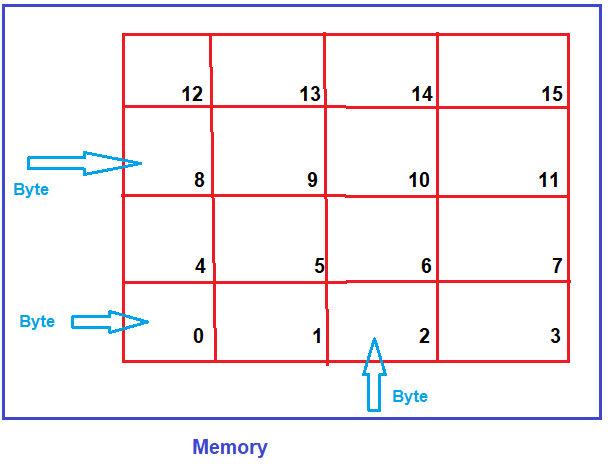##### Memory in Bigger Picture:

Every byte will have its own address. If we take a bigger image of the memory. The below image shows one memory. The corner most byte address is 0 and the upper corner byte’s address is 65536 i.e. total of 0 to 65535 makes 65536 bytes. The total number of bytes is 65536, this is nothing but 64*1024 that is 64 kilobytes. In our entire discussion of this Data Structure and Algorithm course, we will be assuming that the size of the main memory is 64 kilobytes. Nowadays we are using the memory in GB’s like 4GB, 8GB, 16GB memory but to understand we have to take a small part of main memory and that’s why we are taking 64kilobytes of memory,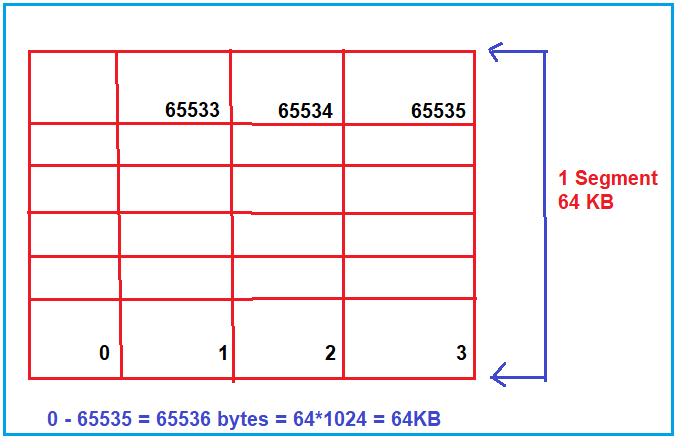As you can see in the above image, the first-byte address is 0 and the last byte address is 65535. This main memory is 64 kilobytes and each byte is having its own address.

In our computers, if we have a larger size of RAM that is 4GB or 8GB or 16GB, then that entire memory is not used as a single unit rather it is divided into manageable pieces called segments and usually the size of a segment will be 64 kilobytes. In our discussion always we will assume that the size of our Main memory is 64KB that is we are talking about a segment.

##### How Program Uses Main Memory?

Now, let us see how our program utilizes the main memory. For better understanding please have a look at the following diagram. Assume that the lowermost block byte address is 0 and the uppermost corner byte address is 65535. As you can see in the below diagram, the entire main memory is divided into three sections (Code section, Stack, and Heap) and used by a program. So, a program uses the main memory by dividing it into three sections i.e. code, stack, and heap.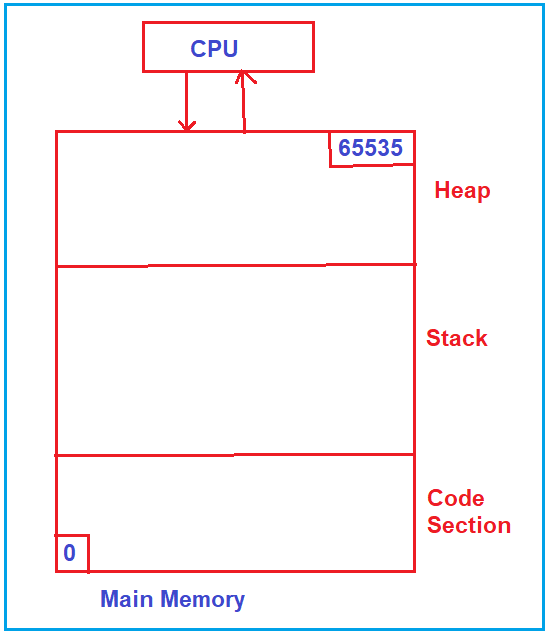Now, let us see how the program utilizes the main memory i.e. the three sections of the main memory. For understanding this please have a look at the below diagram. As you can see on the left-hand side, we have a program on the hard disk. If we want to run this program, then this program i.e. the machine code of the program, first should be brought inside the main memory i.e. brought inside the code section of the Main memory. The area where the machine code of the program is reside called as Code Section of the Main memory. Once the machine code is loaded in the Code Section, then the CPU will start executing the program, and the program then will utilize the remaining memory i.e. stack and heap.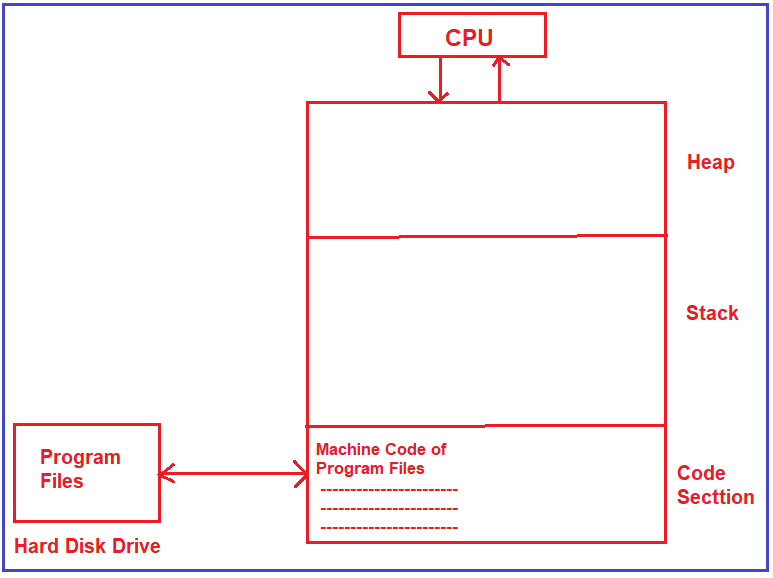##### How does the stack and heap memory work?

Now, let us learn how this stack and heap works. To understand this, we will take one example code and will show you, how the stack memory is used and how heap memory is used. We have taken the following simple example.

void main()
{
int a;
float b;
}

As you can see in the above example, in the main function we have two variables. One is of type integer and the other one is of type float. Now, we assume that integer takes 2 bytes and float takes 4 bytes. In C and C++ programming, the number of bytes taken by an integer depends on the compiler, the operating system, and the hardware but generally we consider compiler. We have two variables (a and b) which take 2 bytes (a variable) and 4 bytes (b variable) i.e. a total of 6 bytes of memory in the program. These 6 bytes of memory are allocated inside the stack and these 6 bytes of memory are given to the program i.e. to the main method which resides in the code section. The block of memory inside the stack which belongs to the main function is called the Stack Frame of Main Function or activation record of the main function. For better understanding, please have a look at the below diagram.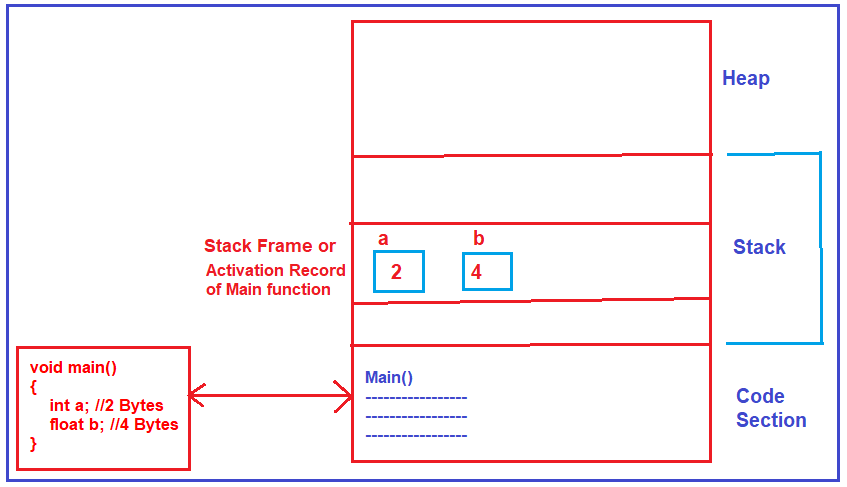Note: The point that you need to remember is whatever variables we declare inside our program or inside a function, the memory for those variables will be allocated inside the stack. So, the portion of memory that is given to the function is called an activation record of that function.

##### What is static memory allocation?

So, how the memory is allocated inside the stack is depends on whatever variables we have inside a function. The size of the memory required by a function was decided at compile-time only by the compiler and that memory is obtained once the program start executing & it is obtained inside the stack, we say this is static memory allocation.

##### What is static here?

How many bytes of memory are required by the function was decided at compile-time, so it is static. When everything is done at compile time or before run time then it is called static.

##### If there is a sequence of function calls then how the memory is allocated inside the stack?

For understanding, please have a look at the below code where we have a sequence of calls. As you can see in the below code, the main function is having two variables (a and b), and then it is calling function fun1(). The function fun1() is having its local variable x and then it is calling fun2() and passing parameter x. The function fun2() is taking parameter as i and also, it’s having its own local variable a. If the following function calls are made then how the memory is allocated for all these functions.

void fun2(int i)
{
int a;
—-
—-
}

void fun1()
{
int x;
fun2();
}

void main()
{
int a;
float b;
—-
—-
fun1();
—-
}

Now, we’ll see how the memory is allocated inside the stack for the above sequence of function calls. First of all, when we run the program, the machine code of the program will be copied in the code section of the Main Memory. When the program starts executing, it will start execution from the main function. The moment it enters inside the main function, it requires a variable. So, the memory for ‘a’ and ‘b’ will be allocated inside the stack area and that section is called the stack frame of the main method or Activation Record of the Main function.

Next, the main function calls function fun1(). That means the control goes inside fun1(). Once the control inside the function fun1, the first thing it required is the variable. And the variable x is created inside the stack and this section is called Stackframe of fun1 function. Now, which function is executing? Currently, fun1() is executing because we have called it & the topmost activation record belongs to which function? Currently executing a function that is fun1().

Then function fun1() calls function fun2(). Once the function fun2 is called, the control goes to fun2(). The function fun2() is having two variables, one is its parameter and the other one is its local variable.

So, the memory is allocated for these variables “i” and “a” inside the stack, and this section is called the Stack Frame of the fun2 function. Now, presently fun2() is running and the topmost activation record inside the stack area is fun2(). For a better understanding of the above-discussed points please have a look at the following image.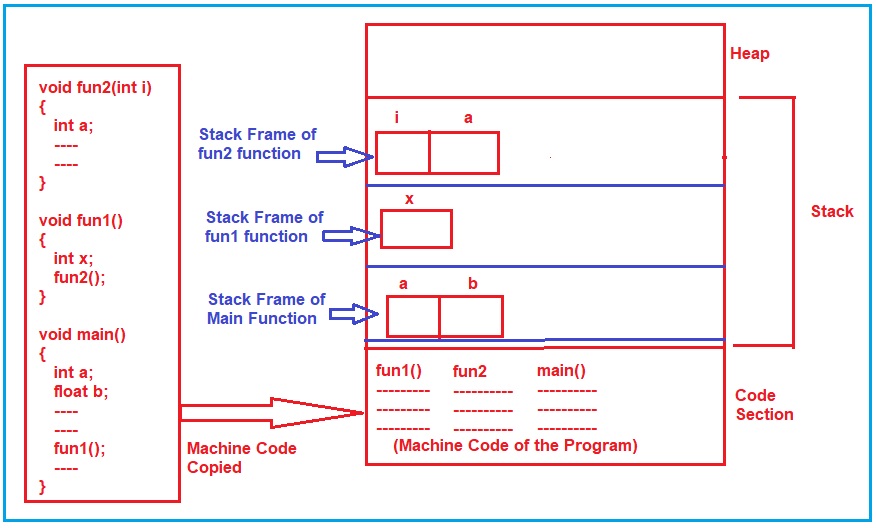One thing that we need to be observed is that we started from the main function and it has not yet finished but it has called fun1(). So, the main function activation record is as it is inside the stack and then the activation record for fun1() is created i.e. memory for fun1() is allocated. then it is still running but it has called function fun2(), So, the activation record for fun2() is created and the activation record of function fun1() is still there in the memory.

Now, let us continue our executing function, when fun2() has finished & terminated, then the control goes back to fun1(). Then what happens to the active record of fun2? This will be deleted. So, once the function fun2 completes its execution and once the control goes back to fun1, then the Action Record or the Stack Frame of the Fun2 function will be deleted from the Stack as shown in the below image.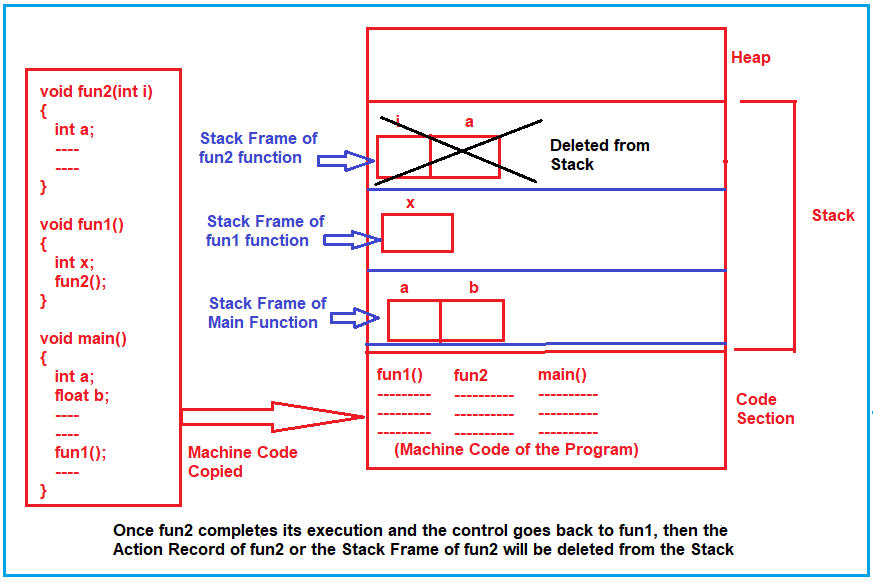Now, let us continue our executing function, when fun1() has finished, then the control again goes back to the Main function and the Action Record or the Stack Frame of Fun2 function will be deleted from the Stack as shown in the below image.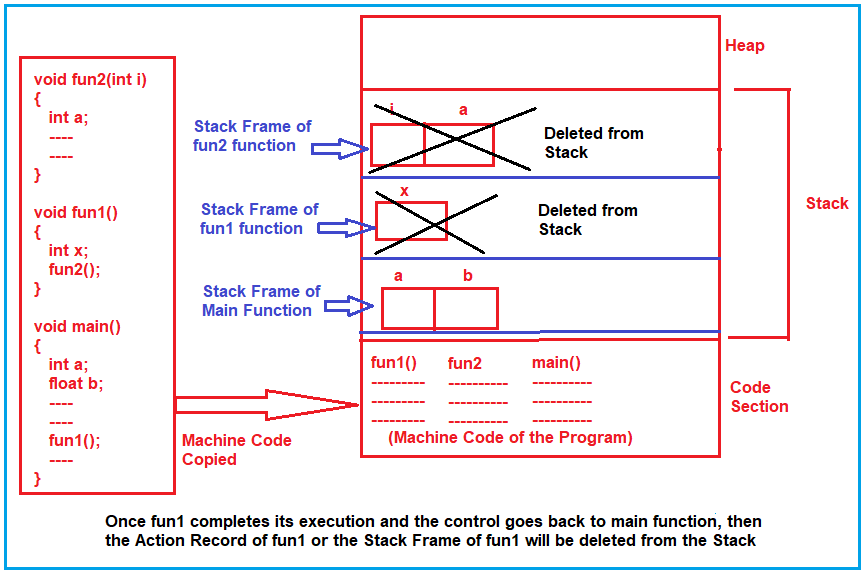Now when the main method completes its execution, its Action Record will also be deleted from the Stack section of the main memory as shown in the below image.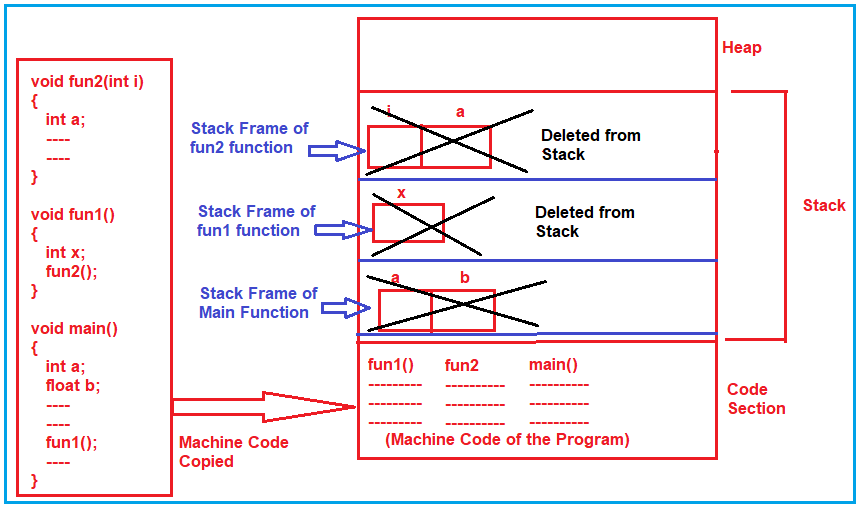This mechanism is called the stack. This section of main memory behaves like a stack during the function call and hence it is named a stack. This is how the main memory is used or stack memory is used for function calls.

##### How much memory is required by a function?

How much memory is required by a function is depends on the number of variables and their sizes and this is decided by the compiler only. The stack memory is automatically created as well as automatically destroyed. The programmer doesn’t have to do anything for its allocation and destruction, just the programmer has to declare the variable. So, the conclusion is whatever the variables we declare in the program or whatever the parameters our functions are taking for all of them, the memory is allocated inside the stack and it is automatically created and automatically destroyed when the function ends.

##### Heap Memory:

Let us learn, how heap memory is utilized by a program? First, let us understand the term Heap. Heap means just piling up, if the things are kept one above another or just randomly, we use the term heap. Heap is used in two cases. One if the things are properly organized like a tower-like thing then also it is a Heap and if it is not organized and also it is looking like a tower then we call it as Heap.

So, the important point that you need to remember is, heap word or term heap can be used for organized things as well as unorganized things. But here heap is the term used for unorganized memory. it is not organized. The stack memory is organized and we already saw how the activation records are created and deleted. This is the first point about heap.

The second point that you need to remember about heap is that heap memory should be treated as a resource. Let us first understand what do you mean by resource. A printer is a resource for your program. If your program wants to use a printer, then it can request a printer and use the printer. Once it has finished using it, it should release the printer. So that the other applications can use it.

In the same way, the heap memory should be used as a resource. When required you take the memory and when you don’t require it, release the memory. This is the practice that we must do while dealing with heap memory.

The third important point is that the program can’t directly access heap memory. The Program can directly access anything inside the code section, anything inside the stack but cannot access the heap memory directly. Then how the program will access the heap memory? It can be access to memory using a pointer.

##### How we can get memory inside the heap with the help of a pointer?

First of all, for taking some memory in heap, we have to take a pointer (int *p). The first question that should come to your mind is how many bytes does a pointer take? In my discussion for making things simple, I say that pointer takes 2 bytes. Actually, the amount of memory taken by the pointer depends on the size of the integer. If the integer size is 2 bytes then the pointer is 2 bytes, if the integer size is 4 bytes then the pointer is 4 bytes. Let us assume that, the integer size is 2 bytes and hence the pointer size is 2 bytes.

Now, the next point where the memory for the pointer will be allocated? It is inside the static memory. We already have seen that all the variables are declared in our functions they will occupy the memory inside the stack in their own activation record. So, for this pointer, the memory will be allocated inside the Stack Frame of Main method or Action Record of Main Method.

Now I want to allocate memory in heap. How much memory do I want to allocate? I want to create an array of integers of size 5 (p = new int). This new statement will allocate memory in the heap of size 5. And the pointer variable will point to the array created in the heap. Suppose, the beginning address of the array is 500 in the heap, then that address (500) will be stored inside the pointer variable p. This is the method of allocating memory in heap. Wherever you see the keyword new, it means the memory is allocated in the heap.

Simple variable declaration memory is allocated inside the stack. In C++, it is the ‘new’ keyword that allocates memory in heap but if I write the same thing in C language then I have to use the malloc() function. For a better understanding of the above discussion, please have a look at the below diagram.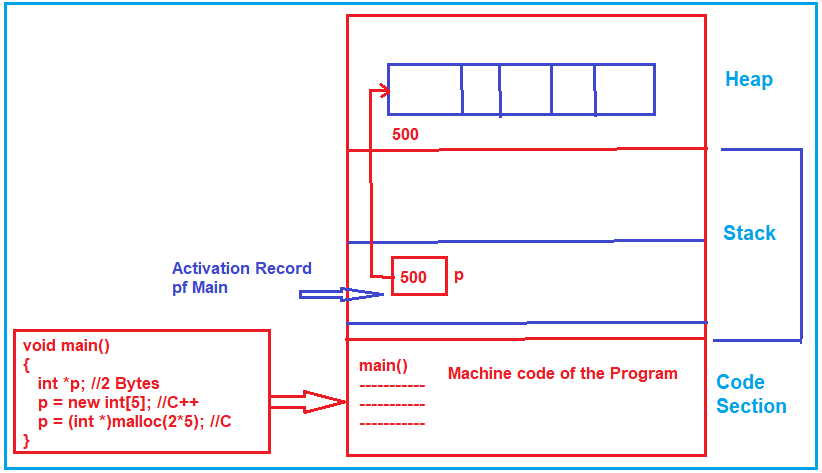##### How the program access heap memory?

The program cannot directly access the heap memory, it has to access the pointer and the pointer will give the address of the heap memory, and then the program can reach that location and access these integers.

One thing I told you at the beginning that the heap memory should be treated as a resource. After some time in your program, if you don’t need that array whose memory is allocated in the heap, then you need to make the pointer “p” as null which means now the pointer will not point to that memory.

Now nothing will be pointing onto that heap memory. Then what about that memory? Is it lost? Is it gone? No, it will not be de-allocated, it is a good practice that when you don’t need the memory, you should de-allocate it (delete []p).

The point that you need to remember is, the heap memory should be explicitly released or disposed. Otherwise, if we are not releasing it, then the memory will be still belonging to our program and that memory cannot be used again. So, it causes loss of memory and the loss of memory is called a memory leak. If we continue the same thing in the program many times then at one stage the heap memory may be full and there will be no free space in heap memory. So, whenever we allocate the memory i.e. heap memory, and if we don’t need it to release the memory.

Let us conclude, we have seen static memory allocation that was done inside the stack for all the variables and then we have seen heap memory allocation, it is done with the help of pointers and when not in use, it must be released. So, finally, we conclude here, we have seen how the static memory allocation is done from the stack and how dynamic memory allocation is done from the heap. This is the difference between stack and heap.

In the next article, I am going to discuss Abstract Data Type (ADT) in detail. Here, in this article, I try to explain Stack vs Heap Memory and I hope you enjoy this Stack vs Heap Memory article. I would like to have your feedback. Please post your feedback, question, or comments about this Static and Dynamic Memory Allocation article.

### 4 thoughts on “Stack vs Heap Memory”

1.I have read many times about this topic that is the difference between stack and heap memory but the way here it’s explained is really great. Everything is so detailed and clearly explained that anyone can understand it very clearly.
Thank you for such a nice article.

2.best content to learn DSA from basic.
please upload all content of topics related to DSA and also C++.

3.Thank you for the clean explanation past 20 years it was a myth for me.

4.Hi, Is dynamic memory allocation means, it will allocate memory at run time in heap?